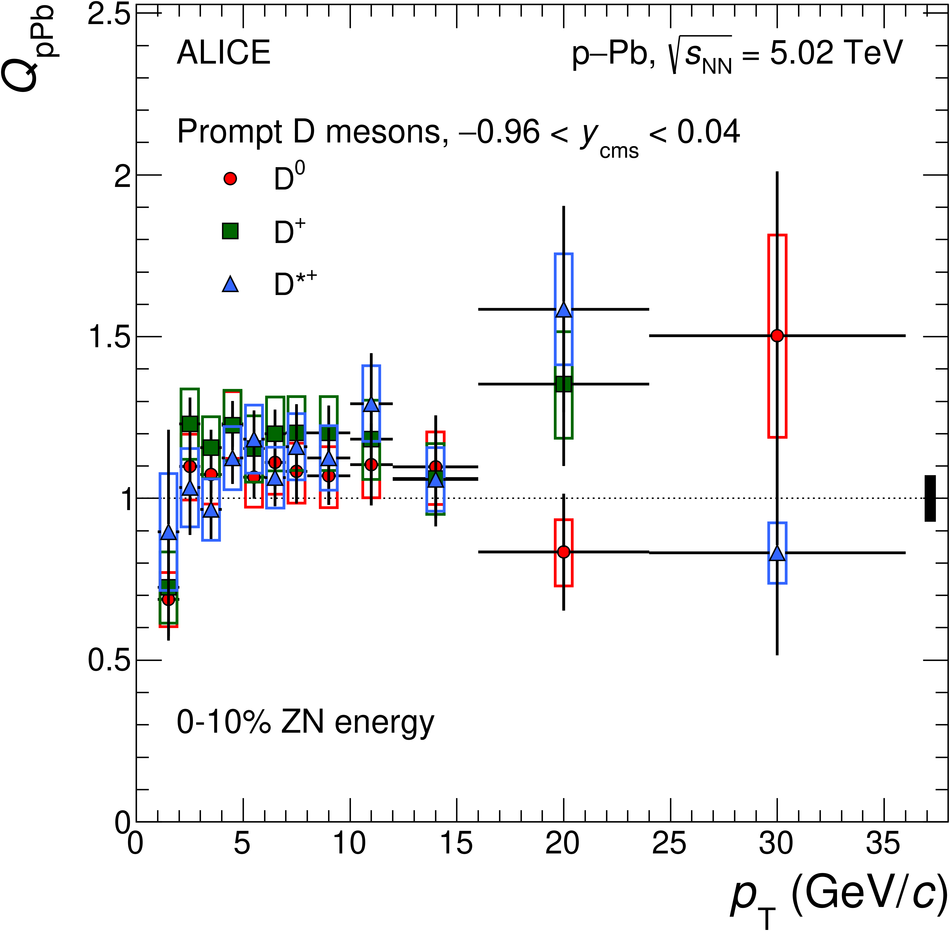# Figure 15

 $\Dzero$, $\Dplus$, and $\Dstar$ meson nuclear modification factors as a function of $\pt$ in the 0--10\% (left) and 60--100\% (right) centrality classes The vertical bars and the empty boxes represent the statistical and systematic uncertainties. The colour-filled boxes at $\QpPb$ $=1$ represent the normalisation uncertainties